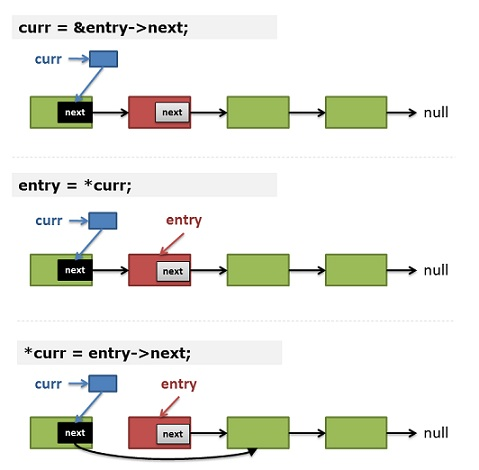## 一重指针

### 简单使用

 ``````1 2 3 4 `````` ``````int a = 1; int *p = &a; //defines an pointer to a, the address where a store. printf("%d", a); printf("%d", *p); //here * is poiner operator, get the value of address = a. ``````

### 数组指针

• 数组名代表数组的首地址，二维数组名则是行地址

• 一维数组

 ``````1 2 3 4 `````` `````` int a = {1, 2, 3, 4}; int *p1 = a; printf("%d", a); //2 printf("%d", (*++p1)); //2 ``````
• 二维（多维）数组

 ``````1 2 3 4 5 6 7 `````` `````` int b = {{1, 2, 3 ,4},{5., 6, 7, 8}}; int (*p2) = b; //**A pointer** to a array of 4 integers,行地址 int (*p3) = &b; //**A pointer** to 2d array b;//5 *((*p2 + 1) + 1); //5,(*p + 1) == 一维数组b的首地址 p2; //5 (*p2); //5 ``````

### 字符串和字符数组

 ``````1 2 3 4 `````` `````` char *s1 = "Hello"; char s2[] = "Hello"; char s3; s3 = "Hello"; //error, assignment to expression with array type ``````
• 赋值方式不同，数组和指针都可以进行初始化，但是数组不能再次进行整体赋值

• 存储内容肯定不一样，数组存储字符串本身，字符型指针存储字符串的首地址

• 指针变量可以改变值（值为地址），数组则不行。

 ``````1 2 `````` `````` s1 += 1; //s1 = "ello" s2 += 1; //error, assignment to expression with array type ``````

### 指针数组

• 字符串指针数组

 ``````1 2 3 `````` ``````char *strings[] = {"Hello", "world!"}; //a array of **two pointers**, which point to a string strings; //Hello strings; //world! ``````

## 二重（多重）指针

 ``````1 2 3 4 5 6 7 8 9 `````` ``````char *strings[] = {"Hello", "world!"}; char *string = "Hello world!"; char **p_string = &string; //a 2d pointer *p_string; //Hello world! char **cur_string = strings; cur_string == *cur_string; //true, Hello cur_string + 1; //world! //it's coool!! ``````

 ``````1 2 3 4 `````` ``````p_string -> string -> "Hello world!" cur_string -> strings -> "Hello" -> "world!" ``````

### 一个编程中遇到的问题

 ``````1 2 3 4 5 6 7 8 9 `````` ``````typedef struct Node { int coeff; int power; struct Node *next; } Polynomial; typedef Polynomial *PtrToNode; typedef PtrToNode Item; typedef PtrToNode Poly; ``````

 `````` 1 2 3 4 5 6 7 8 9 10 11 12 13 14 15 16 17 18 19 20 `````` ``````int comparItem(const void *item1, const void *item2) { Item p1 = (Polynomial *)item1; Item p2 = (Polynomial *)item2; //printf("%d - %d\n", p1->power, p2->power); return (p2->power - p1->power);//from lager to smaller } Poly NewPoly(Item *items, size_t size) { qsort(items, size, sizeof(Item), comparItem); Poly p = malloc(sizeof(Polynomial)); Poly header = p; ZeroPoly(p); for (int i = 0; i < size; i++) { p->next = items[i]; p = p->next; } return header->next; // ignore list header } ``````

Sorting an array of struct pointers using qsort

Your code suggests that you are actually trying sort an array of pointers to struct.

In this case you are missing a level of indirection. You also have your directions reversed.

 ``````1 2 3 4 5 6 `````` ``````//item1(item2) 是指向 Item, 即 (Polynomial *) 的指针。是一个二重指针。 int comparItem(const void *item1, const void *item2) { Item p1 = *(Polynomial **)item1; Item p2 = *(Polynomial **item2; return (p2->power - p1->power); } ``````

### Linus 关于指针的例子

For example, I’ve seen too many people who delete a singly-linked list entry by keeping track of the “prev” entry, and then to delete the entry, doing something like

if (prev) prev->next = entry->next; else list_head = entry->next;

and whenever I see code like that, I just go “This person doesn’t understand pointers”. And it’s sadly quite common.

People who understand pointers just use a “pointer to the entry pointer”, and initialize that with the address of the list_head. And then as they traverse the list, they can remove the entry without using any conditionals, by just doing a “*pp = entry->next”.

Linus 说的 `by just doing a "*pp = entry->next".` 到底是什么意思呢？完整的代码可以看这里

 `````` 1 2 3 4 5 6 7 8 9 10 11 12 13 14 `````` ``````void remove_if(node ** head, remove_fn rm) { for (node** curr = head; *curr; ) { node * entry = *curr; if (rm(entry)) { *curr = entry->next; free(entry); } else curr = &entry->next; } } ``````

• `curr` 是一个指向指针的指针， 也就是 `entry->next` 这个指针的指针。
• 在下一次循环的时候， `entry``*curr`，对二重指针取值，也那么就是 `prev->next`
• 这时如果要删除 `entry`，就把 `curr` 所指的值（也是一个指针）改为 `entry->next`。于是 `prev->next` 就成了 `entry->next`
• 最后 `free` entry。## 函数指针

 `````` 1 2 3 4 5 6 7 8 9 10 11 12 13 14 15 16 `````` ``````int max(int a, int b) { return a > b ? a : b; } int min(int a, int b) { return a > b ? b : a; } int main() { int (*cmp)(int a, int b) = max; printf("Max: %d", cmp(3, 4)); cmp = min; printf("Min: %d", cmp(3, 4)); return 0; } ``````
• 函数指针的定义类似于：`int (*POINTER_NAME)(int a, int b)`， POINTER_NAME 为 指针的名字，指向函数。
• `typedef` 来简化 函数指针的申明：`typedef int (*POINTER_NAME)(int a, int b)`